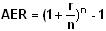# Annual Equivalent Rate (AER)

Financial analysis Print Email

Definition of Annual Equivalent Rate

Annual Equivalent Rate or AER is the rate of interest an investor gets for a fixed deposit for a year on a yearly basis. By definition, Annual Equivalent Rate or AER is a figure which shows what the interest rate on an account would be if interest was paid for a full year and compounded. The interest is calculated to determine the returns that a person can get by adding the interest payment to the amount originally deposited and the next interest payment will be established on the marginally higher account balance.

Formula for Annual Equivalent Rate

The Annual Equivalent Rate or AER can be calculated with the following formula:Where,

n = number of times a year that interest is paid

r = gross rate interest

Explanation of formula with example

AER or Annual Equivalent Rate is compounded annually. If you deposited \$500 in your savings account, at the Annual Equivalent Rate at 5% on your savings, then the balance would be \$525 at the end of the year. The interest amount of \$25 is paid either at the end of every month, or annually.

If the interest is paid more than once in a year the interest is calculated by adding each of the interest payment to the amount originally deposited. If Interest is paid quarterly; the Annual Equivalent Rate or AET will be higher because the gross rate would also have interest paid on the interest itself. AER disregards any bonus interests that are outstanding.

AER or Annual Equivalent Rate is usually confused with APR or Annual Percentage Rate. While Annual Equivalent Rate is calculated on the interest rate on the savings, Annual Percentage Rate is calculated on the borrowings that you make for loans or mortgages etc. AER helps people compare different types of financial products that are available. Annual Equivalent Rate at times can also be confusing as it might not return the actual amount of interest that is charged on the account.

Any rate of interest which is mentioned as Annual Equivalent Rate would be valid if you do not withdraw money from your account during that particular year when the AER is calculated as withdrawing money from your account can affect the overall rate you are expected to receive at the end of the year. Annual Equivalent Rate can be calculated only if you know the frequency of interest payment.